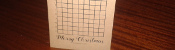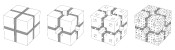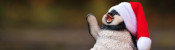mscroggs.co.uk
mscroggs.co.uksubscribe

# Blog

## Christmas card 2017

2017-12-18
Just like last year, TD and I spent some time in November this year designing a puzzle Christmas card for Chalkdust.
The card looks boring at first glance, but contains 10 puzzles. Converting the answers to base 3, writing them in the boxes on the front, then colouring the 1s black and 2s orange will reveal a Christmassy picture.
If you want to try the card yourself, you can download this pdf. Alternatively, you can find the puzzles below and type the answers in the boxes. The answers will be automatically converted to base 3 and coloured...
 # Answer (base 10) Answer (base 3) 1 0 0 0 0 0 0 0 2 0 0 0 0 0 0 0 3 0 0 0 0 0 0 0 4 0 0 0 0 0 0 0 5 0 0 0 0 0 0 0 6 0 0 0 0 0 0 0 7 0 0 0 0 0 0 0 8 0 0 0 0 0 0 0 9 0 0 0 0 0 0 0 10 0 0 0 0 0 0 0
1. In a book with 116 pages, what do the page numbers of the middle two pages add up to?
2. What is the largest number that cannot be written in the form $$14n+29m$$, where $$n$$ and $$m$$ are non-negative integers?
3. How many factors does the number $$2^6\times3^{12}\times5^2$$ have?
4. How many squares (of any size) are there in a $$15\times14$$ grid of squares?
5. You take a number and make a second number by removing the units digit. The sum of these two numbers is 1103. What was your first number?
6. What is the only three-digit number that is equal to a square number multiplied by the reverse of the same square number? (The reverse cannot start with 0.)
7. What is the largest three-digit number that is equal to a number multiplied by the reverse of the same number? (The reverse cannot start with 0.)
8. What is the mean of the answers to questions 6, 7 and 8?
9. How many numbers are there between 0 and 100,000 that do not contain the digits 0, 1, 2, 3, 4, 5, or 6?
10. What is the lowest common multiple of 52 and 1066?

### Similar postsChristmas card 2016Christmas card 2019Christmas card 2018Christmas (2019) is over

Comments in green were written by me. Comments in blue were not written by me.
@Jose: There is a mistake in your answer: 243 (0100000) is the number of numbers between 10,000 and 100,000 that do not contain the digits 0, 1, 2, 3, 4, 5, or 6.
Matthew
Thanks for the puzzle!
Is it possible that the question 9 is no correct?
I get a penguin with perfect simetrie except at answer 9 : 0100000 that breaks the simetry.
Is it correct or a mistake in my answer?
Thx
Jose
@C: look up something called Frobenius numbers. This problem's equivalent to finding the Frobenius number for 14 and 29.
Lewis
I can solve #2 with code, but is there a tidy maths way to solve it directly?
C